# 复杂Gremlin查询的调试方法

【摘要】 Gremlin是图数据库查询使用最普遍的基础查询语言。Gremlin的图灵完备性，使其能够编写非常复杂的查询语句。对于复杂的问题，我们该如何编写一个复杂的查询？以及我们该如何理解已有的复杂查询？本文带你逐步抽丝剥茧，完成复杂查询的调试。

# 1. Gremlin简介

Gremlin是Apache TinkerPop 框架下的图遍历语言。Gremlin是一种函数式数据流语言，可以使得用户使用简洁的方式表述复杂的属性图（property graph）的遍历或查询。每个Gremlin遍历由一系列步骤（可以存在嵌套）组成，每一步都在数据流（data stream）上执行一个原子操作。

Gremlin是一种用于描述属性图中行走的语言。图形遍历分两个步骤进行。

## 1.1. 遍历源（TraversalSource）

Gremlin中的遍历是从TraversalSource开始的。 GraphTraversalSource提供了两种遍历方法。

• GraphTraversalSource.V（Object … ids）：从图形的顶点开始遍历（如果未提供id，则为所有顶点）。
• GraphTraversalSource.E（Object … ids）：从图形的边缘开始遍历（如果未提供id，则为所有边）。

## 1.2. 图遍历（GraphTraversal）

V()和E()的返回类型是GraphTraversal。 GraphTraversal维护许多返回GraphTraversal的方法。GraphTraversal支持功能组合。 GraphTraversal的每种方法都称为一个步骤(step)，并且每个步骤都以五种常规方式之一调制(modulates)前一步骤的结果。

1. map：将传入的遍历对象转换为另一个对象（S→E）。
2. flatMap：将传入的遍历对象转换为其他对象的迭代器（ $S\subseteq E^*$）。
3. filter：允许或禁止遍历器进行下一步（S→S∪∅）。
4. sideEffect：允许遍历器保持不变，但在过程中产生一些计算上的副作用（S↬S）。
5. branch：拆分遍历器并将其发送到遍历中的任意位置（S→{ $S1→E^*，…，S_n→E^*$}→E*）。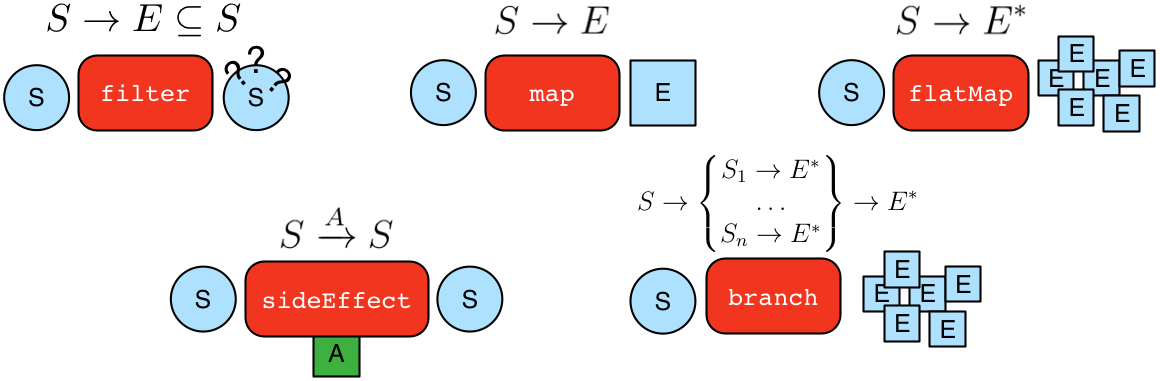• GraphTraversal中几乎每个步骤都从MapStep，FlatMapStep，FilterStep，SideEffectStep或BranchStep扩展得到。

• 举例：找到makro认识的人

gremlin> g.V().has('name','marko').out('knows').values('name')
==>josh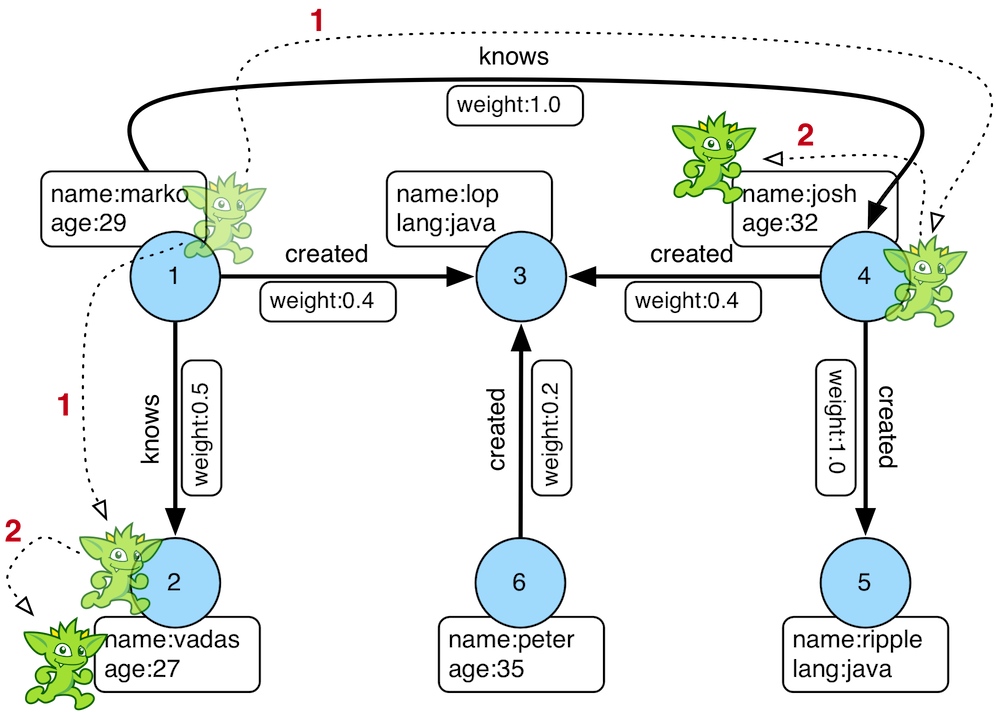# 2. 复杂Gremlin查询的调试

Gremlin的查询都是由简单的查询组合成复杂的查询。所以对于复杂Gremlin查询可以分为以下三个步骤，并逐步迭代完成所有语句的验证，此方法同样适用编写复杂的Gremlin查询。

## 2.1. 迭代调试步骤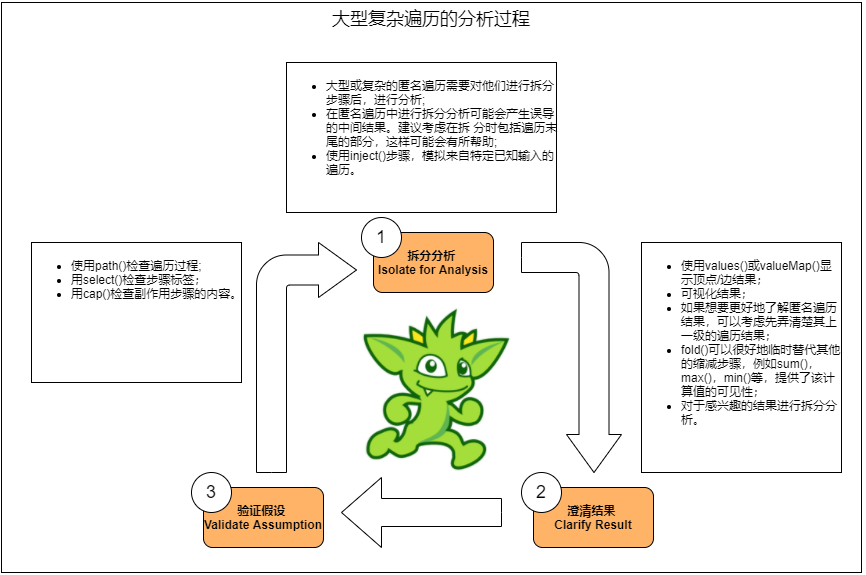1. 拆分分析步骤，划大为小，逐步求证；
2. 输出分步骤的结果，明确步骤的具体输出内容；
3. 对输出结果进行推导和检验。依据结果扩大或缩小分析步骤，回到步骤1继续，直到清楚所有结果。

## 2.2. 用例

### 2.2.1. 图结构

gremlin> graph = TinkerGraph.open()
==>tinkergraph[vertices:0 edges:0]
gremlin> g = graph.traversal()
==>graphtraversalsource[tinkergraph[vertices:0 edges:0], standard]
iterate()
gremlin> graph
==>tinkergraph[vertices:5 edges:5]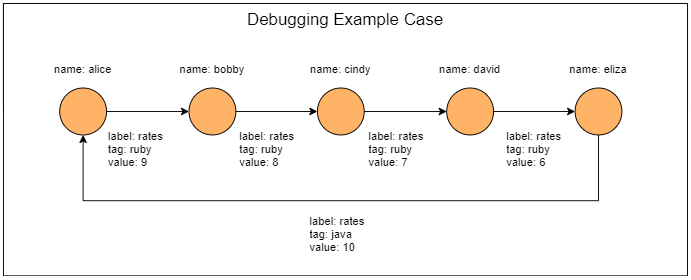### 2.2.2. 查询语句

gremlin>g.V().has('name','alice').as('v').
repeat(outE().as('e').inV().as('v')).
until(has('name','alice')).
store('a').
by('name').
store('a').
by(select(all, 'v').unfold().values('name').fold()).
store('a').
by(select(all, 'e').unfold().
store('x').
by(union(values('value'), select('x').count(local)).fold()).
cap('x').
store('a').by(unfold().limit(local, 1).fold()).unfold().
sack(assign).by(constant(1d)).
sack(div).by(union(constant(1d),tail(local, 1)).sum()).
sack(mult).by(limit(local, 1)).
sack().sum()).
cap('a')
==>[alice,[alice,bobby,cindy,david,eliza,alice],[9,8,7,6,10],18.833333333333332]


## 2.3. 调试过程

### 2.3.1 拆分查询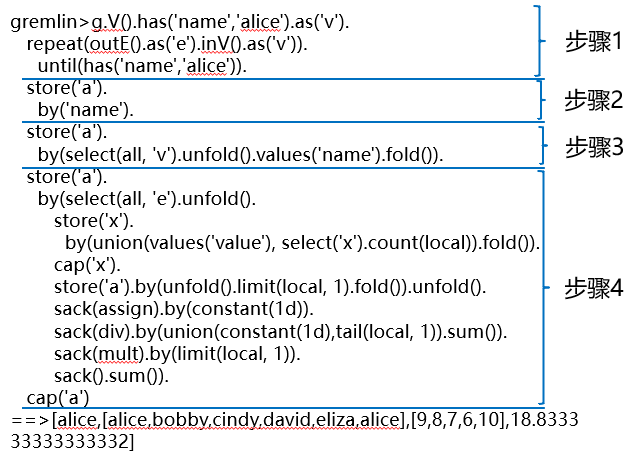• 执行第一部分步骤
gremlin> g.V().has('name','alice').as('v').
......1> repeat(outE().as('e').inV().as('v')).
......2> until(has('name','alice'))
==>v


### 2.3.2 澄清结果

gremlin> g.V().has('name','alice').as('v').
......1> repeat(outE().as('e').inV().as('v')).
......2> until(has('name','alice')).valueMap()
==>[name:[alice]]


### 2.3.3 验证假设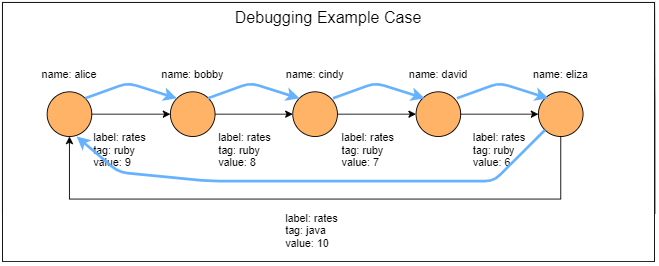g.V().has('name','alice').as('v').
......1> repeat(outE().as('e').inV().as('v')).
......2> until(has('name','alice')).path().next()
==>v
==>e[0-rates->2]
==>v
==>e[2-rates->4]
==>v
==>e[4-rates->6]
==>v
==>e[6-rates->8]
==>v
==>e[8-rates->0]
==>v

• 输出结果与推导结果一致，扩大查询语句, 回到步骤1;
• 如不一致或不理解结果, 缩小步骤范围, 可以采用此步骤的上一层查询步骤,回到步骤1;
• 如此循环直到完全理解整个查询。
gremlin> g.V().has('name','alice').as('v').
......1> repeat(outE().as('e').inV().as('v')).
......2> until(has('name','alice')).
......3> store('a').by('name')
==>v


# 3. 总结

• 在分析的过程，采用划分查询语句的方法，分步理解，采用漏斗式的方法，逐步扩大对语句的理解；
• 对每步的查询结果，可以采用利用valueMap(), path(), select(), as(), cap() 等函数输出和验证结果；
• 对于不清楚结果的步骤或与期望值不一致，缩小查询步骤,可以采用输出步骤的前一步骤作为输出点，进行输出和验证；
• 对于上一层数据的结果明确的情况下，可以采用inject()方式注入上层输出，继续后续的输出和验证；
• 要注意步骤最后的函数，对整个输出结果的影响。

# 4. 参考

【版权声明】本文为华为云社区用户原创内容，转载时必须标注文章的来源（华为云社区），文章链接，文章作者等基本信息，否则作者和本社区有权追究责任。如果您发现本社区中有涉嫌抄袭的内容，欢迎发送邮件至：huaweicloud.bbs@huawei.com进行举报，并提供相关证据，一经查实，本社区将立刻删除涉嫌侵权内容。